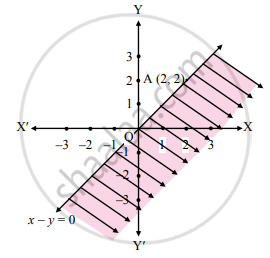# Solve the following inequation graphically in the two-dimensional plane.x – y ≥ 0 - Mathematics and Statistics

Graph

Solve the following inequation graphically in the two-dimensional plane.
x – y ≥ 0

#### Solution

Given, inequation is x – y ≥ 0
∴ Corresponding equation is x – y = 0
It is a line passing through origin O (0, 0)
To draw the line we need one more point.
To find another point on the line, we can take any value of x, Say, x = 2.
∴ substituting x = 2 in x – y = 0, we get
2 – y = 0
∴ y = 2
∴ another point on the line is A (2, 2)
Now origin test is not possible as the origin lies on the line y = x
∴ choose a point which not lie on the line say (3, 1)
∴ substituting x = 3, y = 1 in inequation, we get
3 – 1 ≥ 0
∴ 2 ≥ 0 which is true
∴ all the points on line x − y = 0 and the points on the side where (3, 1) lies satisfy the inequation
The shaded portion represents the solution set.Concept: Graphical Solution of Linear Inequality of Two Variable
Is there an error in this question or solution?
Chapter 8: Linear Inequations - Exercise 8.2 [Page 120]

#### APPEARS IN

Balbharati Mathematics and Statistics 2 (Commerce) 11th Standard HSC Maharashtra State Board
Chapter 8 Linear Inequations
Exercise 8.2 | Q 1. (v) | Page 120
Share